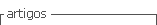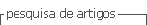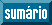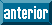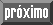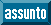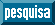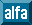## Artigo

•Citado por SciELO
•Acessos

•Citado por Google
•Similares em SciELO
•Similares em Google

## versão impressa ISSN 0120-419X

### Integración - UIS vol.36 no.1 Bucaramanga jan./jun. 2018

#### http://dx.doi.org/10.18273/revint.v36n1-2018005

Artículo Original

A proof of Holsztyński theorem

Una prueba del teorema de Holsztyński

Michael A. Rincón-Villamizar1  marinvil@uis.edu.co

1Universidad Industrial de Santander, Escuela de Matemáticas, Bucaramanga, Colombia.

Abstract

For a compact Hausdorff space, we denote by C(K) the Banach space of continuous functions defined in K with values in R or C. A well known result in Banach spaces of continuous functions is the Holsztyński theorem which establishes that if C(K) is isometric to a subspace of C(S), then K is a continuous image of S. The aim of this paper is to give an alternative proof of this result for extremely regular subspaces of C(K).

MSC2010: 46B03, 46E15, 46E40, 46B25.

Key words: C(K) Banach spaces; Banach-Stone theorem

Resumen

Dado un espacio compacto Hausdorff, denotaremos por C(K) el espacio de Banach de las funciones continuas definidas en K con valores en R o C. Un resultado clasico en la teoria de Espacios de Banach de funciones continuas es el teorema de Holsztyński el cual establece que si C(K) es isometrico a un subespacio de C(S), entonces K es imagen continua de un subespacio de S. El objetivo de este articulo es dar una prueba alternativa de este resultado para subespacios extremadamente regulares de C(K).

Palabras-clave: Espacios de Banach C(K); teorema de Banach-Stone

1. Introduction and main theorems

We will use the standard terminology and notation of Banach space theory. For unexplained definitions and notation we refer to , , , , , , , , , . As usual K stands for the field R or C. For a compact Hausdorff space K, we denote by C(K) the Banach space of K-valued continuous functions on K, provided with the supremum norm.

The classical Banach-Stone theorem states that the Banach space C(K) determines the topology of K , , , . More precisely, if T: C(K) → C(S) is an onto isometry, then there are a homeomorphism h: SK and a continuous function σ: S → K with |σ(s)| = 1 for all sS such that

The conclusion of the Banach-Stone theorem is too far to be valid when we consider into isomorphisms between C(K) spaces. Thus it seems natural to ask for topological properties which are preserved under into isomorphisms of C(K) spaces. In this direction, Holsztyński  proved:

Theorem 1.1. Let K and S be compact Hausdorff spaces. If T: C(K) → C(S) is an into isometry, then there are a closed subset Δ of S, a continuous surjection ψ: Δ → K and a continuous function σ: Δ → K with |σ(s)| = 1 for all s ∈ Δ such that

In , it is established the following generalization of Theorem 1.1 for extremely regular spaces. According to , a closed subspace A of C(K) is called extremely regular if for each kK and each neighborhood U of k and each 0 < ε < 1, there exists fA satisfying ║f║ = f(k) = 1 and |f(w)| < ε for all wK \ U.

Theorem 1.2. Let K and S be compact Hausdorff spaces. Let A be an extremely regular subspace of C(K) and B a closed subspace of C(S). Suppose that T: AB is an into isometry. Then there exist a closed subset Δ of S, a continuous function ψ from Δ onto K and a continuous function σ: Δ → K with |σ(s)| = 1 for all s ∈ Δ such that

The aim of this note is to give an alternative proof of Theorem 1.2. The paper is divided as follows: in the second section we generalize a result which is proved by Plebanek in the setting of C(K) spaces (see [9, Theorem 3.3]). In third section, we prove Theorem 1.2.

2. Preliminaries

Following [7, p. 222], we identify dual space C(K)* with the space of regular countably additive bounded measures, and we denote it by M(K). We always consider M(K) equipped with the weak* topology inherited from C(K)*. The total variation of a measure μM(K) on a Borel set E is denoted by |μ|(E), and its norm by ║μ║ = |μ|(K).

Let K and S be compact Hausdorff spaces. Throughout the paper A denotes an extremely regular subspace of C 0(K). Also B will be a closed subspace of C(S). If sS is fixed and T: AB is an embedding, νs will denote any norm-preserving extension to C(K) of the functional T*δs : A → R defined as T*δs (f) = T f(s) for fA. Also let us assume that T satisfies rf║ ≤ ║T f║ ≤ ║f║ for all fA, where r > 0. Analogously if E = TAB and kK is given, let μk be any norm-preserving extension to C(S) of the functional (T −1)*δk : E → R.

Before stating our first result, we need to establish a notation.

Let kK be given and Vk any fundamental system of open neighborhoods of k. Consider the set Ck = Vk × (0,∞). In Ck we define a partial order as follows: (U, t) ≺ (V, s) iff VU and s < t. Note that (Ck ,≺) is a directed set. It is easy to see that there exists a net (f (U,t))(U,t)∈Ck in A satisfying

1. f (U,t)║ = f (U,t)(k) = 1;

2. |f(U,t)(w)| < t for all wK \ U.

We will write {(U, t), f (U,t)}(U,t)∈Ck ↔ {k} to indicate that the above conditions are satisfied.

Lemma 2.1. Let A be an extremely regular subspace of C(K) and kK given. Suppose that {(U, t), f (U,t)}(U,t)∈Ck ↔ {k}. If μM(K), then

Proof. The statement is obvious if ║μ║ = 0, so we assume that ║μ║ ≠ 0. Let ε > 0 be given. Since |μ| is regular, there is WK open with kW such that |μ|(W\{k}) < ε/2. Let U 0Vk be such that U 0W. If (U 0, ε/2║μ║) ≺ (V, t), we have

The next two results are proved in  for C(K) spaces. However, we noted that they are also valid for extremely regular subspaces of C(K). So, for sake of completeness we include a proof here.

Lemma 2.2. Let kK be fixed. If μ = μk , thenνs ║ ≥ r μ-almost everywhere.

Proof. Let N = {sS: ║δs | E ║ < 1}. We show that μ(N) = 0. For 0 < h < 1, define Nh = {sS: ║δs | E ║ ≤ h}; then Nh is closed and N = ∪ h<1 Nh . It suffices to prove that |μ|(Nh ) = 0 for all h ∈ (0, 1). If ε > 0 is given, then there is fA with ║T f║ ≤ 1 such that ║μ║ − ε < |μ(T f)|. Thus,

Since ║μ║ = |μ|(Nh ) + |μ|(S \ Nh ), we infer that |μ|(Nh ) ≤ ε/1 − h. Thus, |μ|(Nh ) = 0, by the arbitrariness of ε.

Now let sS \ N; then ║δs | E ║ ≥ 1. For a positive number ε there exists fA with ║T f║ ≤ 1 such that |T f(s)| > 1 − ε. From the fact ║f║ ≤ 1/r, we infer that r(1 − ε) < ║νs ║. So, the result follows when ε → 0. ☑

If h is a real valued function defined on a topological space X, the oscillation of h at x on a set A is

where the infimum is taken over all open neighborhoods U of x.

Lemma 2.3. Let kK and ε > 0 be fixed. Consider the measure μ = μk. Suppose that there is a compact subset F of S such that

1. νs ║ ≥ r for all sF;

2. osc s (║νs ║, F) ≤ ε for all sF;

3. |μ|(S \ F) < ε.

Then, there is sF such that |νs ({k})| ≥ r − 2ε.

Proof. Let δ > 0 be given and let UK be open with kK. Since A is extremely regular, there exists fU A such that ║fU ║ = fU (k) = 1 and |fU (w)| < δ for all wK \ U. We will show that there is sU F satisfying |T fU (sU )| > rε. Indeed, if |T fU (s)| < rε for all sF, then

which is absurd. Now if sU F satisfies |T fU (sU )| > rε, then

since ║νsU ║ = ║T *δsU ║ ≤ 1. So if δ → 0, then rε ≤ |νsU |(U). Let Vk be a fundamental system of open neighborhoods of k and consider the net (sU ) U Vk in F. Since F is compact, there is a subnet (sU ) UW converging to sF. By (2), so we may assume that ║νsU ║ ≤ ║νs ║ + ε for all UW.

Now, if UK is open with kU, then we have |νs |(U) ≥ r − 2ε. Indeed, by Urysohn Lemma [7, Proposition 4.32] there exists g: K → [0, 1] continuous such that g = 1 on an open set V containing k and g = 0 outside U. Thus, if WW satisfies WV , then |υsW |(g) ≥ |υsW |(W) ≥ rε. Whence,

Since νsW νs in the weak* topology, by [9, Lemma 2.1] and the above inequality we have

Therefore, |νs |(U) ≥ |νs |(g) ≥ r − 2ε. Regularness of νs implies |νs ({k})| ≥ r − 2ε, and the proof is complete. ☑

The proof of the next result follows as in [9, Theorem 3.3] by using Lemmas 2.2 and 2.3.

Theorem 2.4. Let K and S be compact Hausdorff spaces. Suppose that T : AB is an embedding. For each kK we have

3. Proof of Theorem 1.2

Since T is an isometry we have ║T ║ = ║T −1║ = 1. For kK we set

By Theorem 2.4 we have Δ k ≠ ∅ for each kK.

Claim 3.1. If k 1, k 2K and k 1k 2, then Δ k ∩ Δ k = ∅.

If not, let sS be such that s ∈Δ k ∩ Δ k . Then

By taking a, bK with aT*δs ({k 1}) = 1 and bT *δs ({k 2}) = 1, we infer from definition of variation that

which is absurd. This proves the claim.

Claim 3.2. Let kK be given. If s ∈ Δ k , then there is as K with |as | = 1 such that T f(s) = asf(k) for all fA.

Indeed, if s ∈ Δ k , then as = T *δs ({k}) ∈ K and |as | = 1. On the other hand, T *δs = asδk + μ, where μM(K) satisfies μ({k}) = 0. So, it follows that

So, ║μ║ = 0, which means that μ = 0. Hence T *δs = asδk , that is, T f(s) = asf(k) for all fA, as claimed.

Set Δ = ∪ k∈K Δ k , and let ψ: Δ → K and σ: Δ → K be defined as ψ(s) = k and σ(s) = as , respectively, iff s ∈ Δ k , where as is determined as in Claim 3.2. Note that ψ is well-defined by Claim 3.1. The surjectivity of ψ is consequence from the fact Δ k ≠ ∅ for each kK. Clearly, |σ(s)| = 1 for all sS. Also, by Claim 3.2 we have

Claim 3.3. ψ: Δ → K and σ: Δ → K are continuous.

Let s ∈ Δ be given and (sα ) a net in Δ such that sα s. Suppose that ψ(sα ) = kα ψ(s) = k. Thus, there is a compact neighborhood VK of k such that for all α, there is α α with V . Since A is extremely regular, there exists fA such that ║f║ = f(k) = 1 and |f(w)| < 1/2 for all wK \ V . Note that |T f(s)| = |f(ψ(s))| = |f(k)| = 1. By continuity of T f, there is α0 such that |T f(sα )| > 1/2 for all αα 0. By taking α α 0 with kα′ V , we have 1/2 > |f(kα )| = |f(ψ(sα ))| = |T f(sα )| > 1/2, which is impossible.

Now we prove continuity of σ. Let s ∈ Δ be given and ψ(s) = k. Take fA such that ║f║ = f(k) = 1. By Equation (2) we have σ(s) = T f(s), and continuity follows immediately.

Claim 3.4. Δ is closed.

Let (sα ) be a net in Δ and suppose that sα s for some sS. Write ψ(sα ) = kα for all α. By compactness of K, we may assume that kα k for some kK. By Claim 3.2 we have |T f(sα )| = |f(ψ(sα ))| = |f(kα )| for all fA. Thus, |T f(s)| = |f(k)| for all fA. Let (f (U,t))(U,t)∈Ck be a net in A such that {(U, t), f (U,t)}(U,t)∈ Ck ↔ {k}. Then |T f (U,t)(s)| = |f (U,t)(k)| = 1 for all (U, t) ∈ Ck . Once again by Lemma 2.1, we have

So, |T *δs ({k})| = 1, that is, s ∈ Δ.

References

 Albiac F. and Kalton N.J., Topics in Banach space theory, Graduate Texts in Mathematics 233, Springer, New York, 2006. [ Links ]

 Araujo J., Font J.J. and Hernandez S., “A note on Holsztynski’s theorem”, Papers on general topology and applications, in Ann. New York Acad. Sci. 788, New York (1996), 9–12. [ Links ]

 Arens R.F. and Kelley J.L., “Characterization of the space of continuous functions over a compact Hausdorff space”, Trans. Amer. Math. Soc. 62 (1947), 499–508. [ Links ]

 Banach S., Théorie des opérations linéaires, Monografie Matematyczne, Warsaw, 1933. [ Links ]

 Behrends E., M-Sctructure and Banach-Stone theorem, Lecture Notes in Math. 736, Springer-Verlag, 1979. [ Links ]

 Cengiz B., “Continuous maps induced by isomorphisms of extremely regular function spaces”, J. Pure Appl. Sci. 18 (1985), No. 3, 377–384. [ Links ]

 Folland G.B., Real analysis, Modern techniques and their applications, Pure and Applied Mathematics (New York), A Wiley-Interscience Publication, John Wiley & Sons Inc., New York, 1999. [ Links ]

 Holsztyński W., “Continuous mappings induced by isometries of spaces of continuous functions”, Studia Math. 26 (1966), 133–136. [ Links ]

 Plebanek G., “On isomorphisms of Banach spaces of continuous functions”, Israel J. Math. 209 (2015), No. 1, 1–13. [ Links ]

 Semadeni Z., Banach spaces of Continuous Functions, Vol. 1, Monografie Mat. 55, PWN-Polish Sci. Publ. Warszawa, 1971. [ Links ]

 Stone M.H., “Applications of the theory of Boolean rings to general topology”, Trans. Amer. Math. Soc. 41 (1937), 375–481. [ Links ]

Received: May 17, 2018; Accepted: June 29, 2018This work is under a Creative Commons Attribution 4.0 International License.